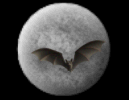General Forum Message Forums: Atm · Astrophotography · Blackholes · Blackholes2 · CCD · Celestron · Domes · Education Eyepieces · Meade · Misc. · God and Science · SETI · Software · UFO · XEphemBe the first pioneers to continue the Astronomy Discussions at our new Astronomy meeting place...The Space and Astronomy Agora I'll Try Forum List | Follow Ups | Post Message | Back to Thread Topics | In Response ToPosted by Bruce on March 22, 2001 21:30:50 UTC

In relativity an objects natural motion is described by Newton's first law of motion. This is called a geodesic. For the gravitiational field this is a straight line through curved spacetime. This is an objects natural motion and is also referred to as free fall. There is no force of gravity in GR. For a freely falling object there are three constants of the motion. Energy per unit mass E/m, momentum per unit mass p/m (in SR) and angular momentum per unit mass L/m. The Schwarzchild metric was the first solution to Einstein's equations. Even though this metric is written for describing spacetime around spherically symmetric non-rotating bodies of mass it is still a very good approximation for slowly spinning objects such as the Sun and planets of our solar system. From the Schwarzchild metric these constants of motion are derived
E/m-(1-2M/r)dt/dt
so
dt=[(E/m)/(1-2M/r)]dt
L/m=r2df/dt
so
df=[(L/m)/r2]dt
Substitute dt and df into the Schwarzchild metric and solve for dr and you get this expression for computing the orbit
dr= + or - [(E/m)2 - (1-2M/r){1 + (L/m)2}]1/2dt
Basically all this means is the energy and angular momentum per unit mass is conserved regardless of the geometry of the orbit. The orbiting object follows its natural motion through spacetime with no forces acting upon it. There is some more about this at the following site
http://www.physics.purdue.edu/~hinson/ftl/html/FTL_part3.html
Scroll down to equation 5:69 (which is the same equation expressed slightly different) and read the text below the equation.